## A 2.26 cm tall object is placed in 18.6 cm in front of a convex lens. The focal length is 35.8 cm. How far is the image from the lens?

Question

A 2.26 cm tall object is placed in 18.6 cm in front of a convex lens. The focal length
is 35.8 cm. How far is the image from the lens?

in progress 0
2 weeks 2021-08-30T01:26:31+00:00 1 Answers 0 views 0

the image is -38.7 cm from the lens

Explanation:

We use the lens equation which says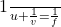where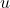is the distance to the object,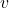is the distance to the image, and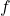is the focal length.

Now, in our case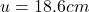,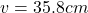,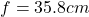;

therefore,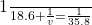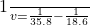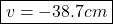where the negative sign indicates that the image is virtual and on the same side of the lens as the object.